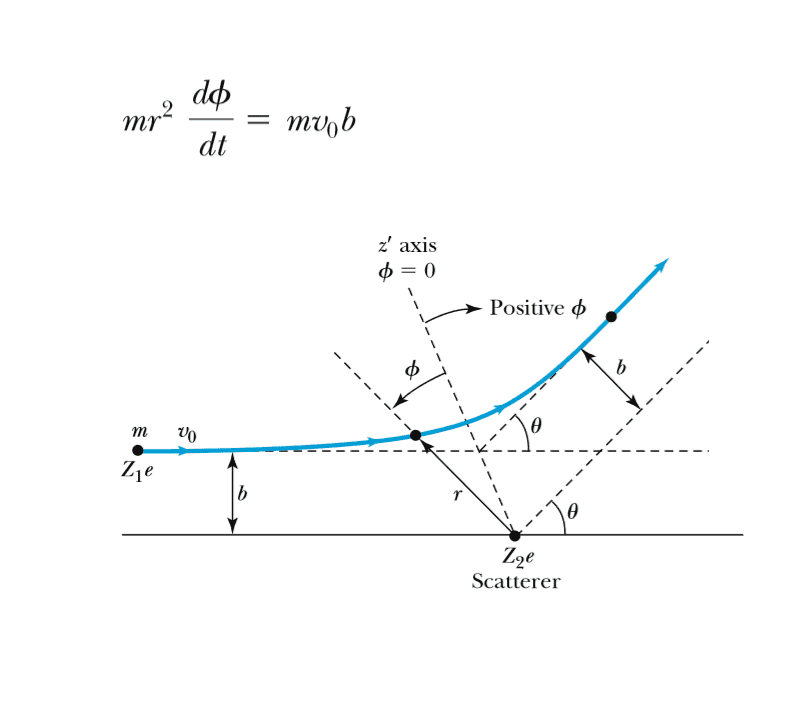# Law of conservation of angular momentum

Boltzman Oscillation
Given the figure, how can i arrive to this formula knowing that angular momentum is conserved?I know that p = mv and L = p x r. So the initial momentum will be L1 = mV x R and the final momentum will be L2 = mv x r.

I am not sure how R will equal to b since the distance between the initial position of the electron is clearly not b distance apart from the scatterer. I am also not sure how to modify the final angular momentum to fit the formula.

#### Attachments

I know that p = mv and L = p x r. So the initial momentum will be L1 = mV x R and the final momentum will be L2 = mv x r.

I am not sure how R will equal to b since the distance between the initial position of the electron is clearly not b ...
Your b is the magnitude of the r vector component, that is perpendicular to p (or v), before and after the scattering. Do you understand the cross product?
https://en.wikipedia.org/wiki/Cross_product

Last edited:
Boltzman Oscillation
Your b is the magnitude of the r vector component, that is perpendicular to p (or v), before and after the scattering. Do you understand the cross product?
https://en.wikipedia.org/wiki/Cross_product
yes I understand the cross product but I've not had enough practice with it (my math foundations class was a mess). Isnt the magnitude how large the arrow is? In that case then the magnitude b wouldn't be the same as the magnitude of vector r would it? r stretches and shortens throughout the path of movement.

In that case then the magnitude b wouldn't be the same as the magnitude of vector r would it?
Look at your picture. Do they look the same?

r stretches and shortens throughout the path of movement.
Yes, but b is just one component of r, before and after scattering, not throughout.

Boltzman Oscillation
Look at your picture. Do they look the same?

Yes, but b is just one component of r, before and after scattering, not throughout.
ohh so I am only looking at before and after the scattering and not throughout. Hmm so in that case r = b in both cases. Now the velocity of the particle after the scattering can be described by position/time. Well position will be given by the angle. The derivative of position in respect to time is position right? So the velocity will equal d(angle)/dt. The final angular momentum will then equal:

L = m * d(angle)/dt x b. = m*(dΦ/dt)*bsin(90) = m*(dΦ/dt)?

Ugh why dint I learn vector calculus correctly? :(
I guess its best to take my time to learn it now so it won't impede me later.

b is just one component of r
r = b
No, see above.

Boltzman Oscillation
No, see above.
Okay so L = m*(dΦ/dt) x r = m*(dΦ/dt)*b*sin(angle between the two) ?

Okay so L = m*(dΦ/dt) x r = m*(dΦ/dt)*b*sin(angle between the two) ?
Which is greater, b or |r|?

Boltzman Oscillation
Which is greater, b or |r|?
err I thought b was the magnitude of r? so arent they the same in errrr greatness?

Boltzman Oscillation
err I thought b was the magnitude of r? so arent they the same in errrr greatness?
sigh, on the initial momentum then b will be the yth component of r while in the final momentum r will be greater than b.